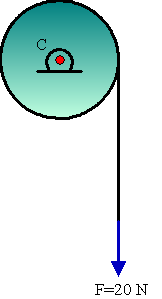Visit the website 123iitjee.manishverma.site for latest posts, courses, admission & more.

###Consider a 20 kg uniform circular disk of radius 0.2 m. It is pin supported at its centre and is at rest initially. The disk is acted upon by a constant force F = 20 N through a massless string wrapped around its periphery as shown in the figure. Suppose the disk makes n number of revolutions to attain an angular speed of 50 rad/s. The value of n, to the nearest integer, is ---------. [Given : In one complete revolution, the disk rotates by 6.28 rad]

Solution

We have, $\omega ^2 = 2\alpha \theta$

Or, $\omega ^2 = 2\alpha .(n.2\pi)$

$\Rightarrow n = \frac {\omega ^2}{4\pi \alpha }$

Torque $\tau = rF = I\alpha$

$\Rightarrow \alpha = \frac {rF}{I}$

So, $n = \frac {I \omega ^2}{4\pi rF}=\frac {mr^2 \omega ^2}{8\pi rF}=\frac {mr \omega ^2}{8\pi F}=\frac {20\times 0.2 \times 50^2}{4\times 6.28 \times 20}$

$\Rightarrow n=19.9 \approx 20$